• 图像中，已知了某连通域的一个像素点，如何根据该像素点确定像素点所在的连通域(比如图像中有多个连通域，而现在只知一个连通域像素点，如何根据该点反推像素点所在的连通域，并标记出来)图像处理 算法
• 图像处理（五）——连通域

万次阅读 2018-11-07 22:35:33
连通区域（Connected Component）一般是指图像中具有相同像素值且位置相邻的前景像素点组成的图像区域（Region，Blob）。连通区域分析（Connected Component Analysis,Connected Component Labeling）是指将图像中的...

连通区域（Connected Component）一般是指图像中具有相同像素值且位置相邻的前景像素点组成的图像区域（Region，Blob）。连通区域分析（Connected Component Analysis,Connected Component Labeling）是指将图像中的各个连通区域找出并标记。
连通区域分析是一种在CVPR和图像分析处理的众多应用领域中较为常用和基本的方法。例如：OCR识别中字符分割提取（车牌识别、文本识别、字幕识别等）、视觉跟踪中的运动前景目标分割与提取（行人入侵检测、遗留物体检测、基于视觉的车辆检测与跟踪等）、医学图像处理（感兴趣目标区域提取）、等等。也就是说，在需要将前景目标提取出来以便后续进行处理的应用场景中都能够用到连通区域分析方法，通常连通区域分析处理的对象是一张二值化后的图像。

而这次我要做的是实现图像的快速连通域算法，可以提取出图像中的连通域，并将不同连通域用不同颜色表示。

寻找图像中的连通域的算法有两个，一个是Two-Pass方法

Two-Pass算法的简单步骤：

（1）第一次扫描：
访问当前像素B(x,y)，如果B(x,y) == 1：

a、如果B(x,y)的领域中像素值都为0，则赋予B(x,y)一个新的label：
label += 1， B(x,y) = label；

b、如果B(x,y)的领域中有像素值 > 1的像素Neighbors：
1）将Neighbors中的最小值赋予给B(x,y):
B(x,y) = min{Neighbors}

2）记录Neighbors中各个值（label）之间的相等关系，即这些值（label）同属同一个连通区域；

labelSet[i] = { label_m, …, label_n }，labelSet[i]中的所有label都属于同一个连通区域

图示为：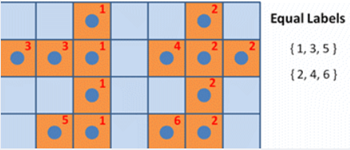// 1. 第一次遍历

_lableImg.release();
_binImg.convertTo(_lableImg, CV_32SC1);

int label = 1;  // start by 2
std::vector<int> labelSet;
labelSet.push_back(0);   // background: 0
labelSet.push_back(1);   // foreground: 1

int rows = _binImg.rows - 1;
int cols = _binImg.cols - 1;
for (int i = 1; i < rows; i++)
{
int* data_preRow = _lableImg.ptr<int>(i - 1);
int* data_curRow = _lableImg.ptr<int>(i);
for (int j = 1; j < cols; j++)
{
if (data_curRow[j] == 1)
{
std::vector<int> neighborLabels;
neighborLabels.reserve(2);
int leftPixel = data_curRow[j - 1];
int upPixel = data_preRow[j];
if (leftPixel > 1)
{
neighborLabels.push_back(leftPixel);
}
if (upPixel > 1)
{
neighborLabels.push_back(upPixel);
}

if (neighborLabels.empty())
{
labelSet.push_back(++label);  // assign to a new label
data_curRow[j] = label;
labelSet[label] = label;
}
else
{
std::sort(neighborLabels.begin(), neighborLabels.end());
int smallestLabel = neighborLabels;
data_curRow[j] = smallestLabel;

// save equivalence
for (size_t k = 1; k < neighborLabels.size(); k++)
{
int tempLabel = neighborLabels[k];
int& oldSmallestLabel = labelSet[tempLabel];
if (oldSmallestLabel > smallestLabel)
{
labelSet[oldSmallestLabel] = smallestLabel;
oldSmallestLabel = smallestLabel;
}
else if (oldSmallestLabel < smallestLabel)
{
labelSet[smallestLabel] = oldSmallestLabel;
}
}
}
}
}
}

（2）第二次扫描：
访问当前像素B(x,y)，如果B(x,y) > 1：

a、找到与label = B(x,y)同属相等关系的一个最小label值，赋予给B(x,y)；
完成扫描后，图像中具有相同label值的像素就组成了同一个连通区域。

图示为：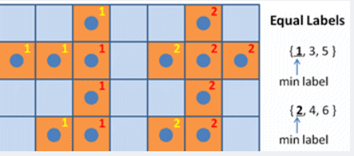// 2. 第二遍扫描
for (int i = 0; i < rows; i++)
{
int* data = _lableImg.ptr<int>(i);
for (int j = 0; j < cols; j++)
{
int& pixelLabel = data[j];
pixelLabel = labelSet[pixelLabel];
}
}
}

另一个方法就是Seed-Filling方法

种子填充法的连通区域分析方法：

（1）扫描图像，直到当前像素点B(x,y) == 1：

a、将B(x,y)作为种子（像素位置），并赋予其一个label，然后将该种子相邻的所有前景像素都压入栈中；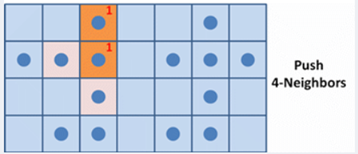b、弹出栈顶像素，赋予其相同的label，然后再将与该栈顶像素相邻的所有前景像素都压入栈中；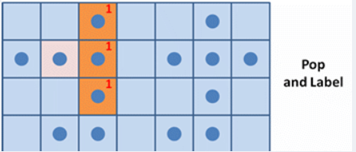// 推及到四个邻居
if (_lableImg.at<int>(curX, curY - 1) == 1)
{// 左边的像素
neighborPixels.push(std::pair<int, int>(curX, curY - 1));
}
if (_lableImg.at<int>(curX, curY + 1) == 1)
{// 右边的像素
neighborPixels.push(std::pair<int, int>(curX, curY + 1));
}
if (_lableImg.at<int>(curX - 1, curY) == 1)
{// 上面的像素
neighborPixels.push(std::pair<int, int>(curX - 1, curY));
}
if (_lableImg.at<int>(curX + 1, curY) == 1)
{// 下面的像素
neighborPixels.push(std::pair<int, int>(curX + 1, curY));
}

c、重复b步骤，直到栈为空；
此时，便找到了图像B中的一个连通区域，该区域内的像素值被标记为label；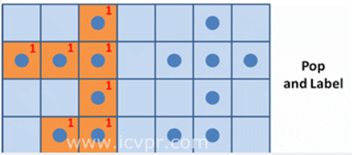（2）重复第（1）步，直到扫描结束；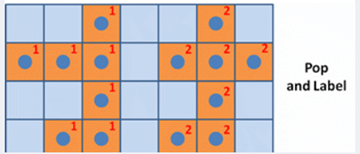扫描结束后，就可以得到图像B中所有的连通区域；

而我选择的是种子填充方法。
对下面这张图片做处理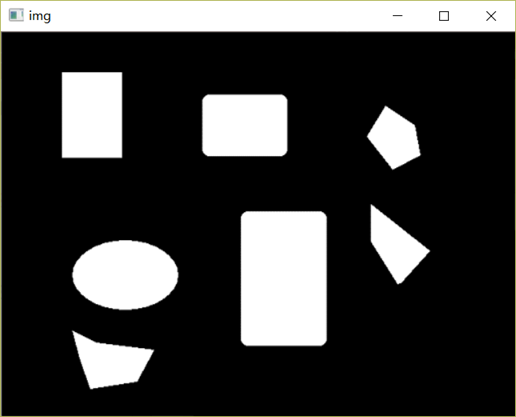得到结果为：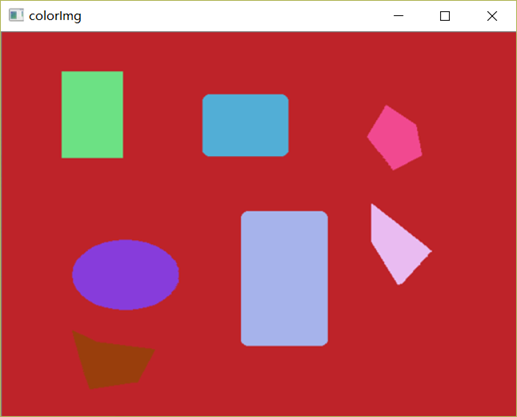改变连通域颜色：

我用的方法是在刚开始的时候就随机设置三个RGB 值，然后为填充不同连通域（每个连通域的像素的RGB值都是随机的）。

cv::Scalar icvprGetRandomColor()
{
uchar r = 255 * (rand() / (1.0 + RAND_MAX));
uchar g = 255 * (rand() / (1.0 + RAND_MAX));
uchar b = 255 * (rand() / (1.0 + RAND_MAX));
return cv::Scalar(b, g, r);
}

同时也欢迎各位关注我的微信公众号 南木的下午茶代码自取

// CVE6.cpp: 定义控制台应用程序的入口点。
//

#include "stdafx.h"
#include <iostream>
#include <string>
#include <list>
#include <vector>
#include <map>
#include <stack>
#include <opencv2/imgproc/imgproc.hpp>
#include <opencv2/highgui/highgui.hpp>
#include <stdio.h>
using namespace cv;
//Two Pass方法
void icvprCcaByTwoPass(const cv::Mat& _binImg, cv::Mat& _lableImg)
{
/*
Two-Pass算法的简单步骤：
（1）第一次扫描：
访问当前像素B(x,y)，如果B(x,y) == 1：
a、如果B(x,y)的领域中像素值都为0，则赋予B(x,y)一个新的label：
label += 1， B(x,y) = label；
b、如果B(x,y)的领域中有像素值 > 1的像素Neighbors：
1）将Neighbors中的最小值赋予给B(x,y):
B(x,y) = min{Neighbors}
2）记录Neighbors中各个值（label）之间的相等关系，即这些值（label）同属同一个连通区域；
labelSet[i] = { label_m, .., label_n }，labelSet[i]中的所有label都属于同一个连通区域
（2）第二次扫描：
访问当前像素B(x,y)，如果B(x,y) > 1：
a、找到与label = B(x,y)同属相等关系的一个最小label值，赋予给B(x,y)；
完成扫描后，图像中具有相同label值的像素就组成了同一个连通区域。
*/

if (_binImg.empty() ||
_binImg.type() != CV_8UC1)
{
return;
}

// 1. 第一次遍历

_lableImg.release();
_binImg.convertTo(_lableImg, CV_32SC1);

int label = 1;  // start by 2
std::vector<int> labelSet;
labelSet.push_back(0);   // background: 0
labelSet.push_back(1);   // foreground: 1

int rows = _binImg.rows - 1;
int cols = _binImg.cols - 1;
for (int i = 1; i < rows; i++)
{
int* data_preRow = _lableImg.ptr<int>(i - 1);
int* data_curRow = _lableImg.ptr<int>(i);
for (int j = 1; j < cols; j++)
{
if (data_curRow[j] == 1)
{
std::vector<int> neighborLabels;
neighborLabels.reserve(2);
int leftPixel = data_curRow[j - 1];
int upPixel = data_preRow[j];
if (leftPixel > 1)
{
neighborLabels.push_back(leftPixel);
}
if (upPixel > 1)
{
neighborLabels.push_back(upPixel);
}

if (neighborLabels.empty())
{
labelSet.push_back(++label);  // assign to a new label
data_curRow[j] = label;
labelSet[label] = label;
}
else
{
std::sort(neighborLabels.begin(), neighborLabels.end());
int smallestLabel = neighborLabels;
data_curRow[j] = smallestLabel;

// save equivalence
for (size_t k = 1; k < neighborLabels.size(); k++)
{
int tempLabel = neighborLabels[k];
int& oldSmallestLabel = labelSet[tempLabel];
if (oldSmallestLabel > smallestLabel)
{
labelSet[oldSmallestLabel] = smallestLabel;
oldSmallestLabel = smallestLabel;
}
else if (oldSmallestLabel < smallestLabel)
{
labelSet[smallestLabel] = oldSmallestLabel;
}
}
}
}
}
}

// 更新标签
// 用每个连通域中最小的标签来表示这个连通域
for (size_t i = 2; i < labelSet.size(); i++)
{
int curLabel = labelSet[i];
int preLabel = labelSet[curLabel];
while (preLabel != curLabel)
{
curLabel = preLabel;
preLabel = labelSet[preLabel];
}
labelSet[i] = curLabel;
}

// 2. 第二遍扫描
for (int i = 0; i < rows; i++)
{
int* data = _lableImg.ptr<int>(i);
for (int j = 0; j < cols; j++)
{
int& pixelLabel = data[j];
pixelLabel = labelSet[pixelLabel];
}
}
}

void icvprCcaBySeedFill(const cv::Mat& _binImg, cv::Mat& _lableImg)
{
/*
种子填充法的连通区域分析方法：
（1）扫描图像，直到当前像素点B(x,y) == 1：
a、将B(x,y)作为种子（像素位置），并赋予其一个label，然后将该种子相邻的所有前景像素都压入栈中；
b、弹出栈顶像素，赋予其相同的label，然后再将与该栈顶像素相邻的所有前景像素都压入栈中；
c、重复b步骤，直到栈为空；
此时，便找到了图像B中的一个连通区域，该区域内的像素值被标记为label；
（2）重复第（1）步，直到扫描结束；
扫描结束后，就可以得到图像B中所有的连通区域；
*/

if (_binImg.empty() ||
_binImg.type() != CV_8UC1)
{
return;
}

_lableImg.release();
_binImg.convertTo(_lableImg, CV_32SC1);

int label = 1;  // start by 2

int rows = _binImg.rows - 1;
int cols = _binImg.cols - 1;
for (int i = 1; i < rows - 1; i++)
{
int* data = _lableImg.ptr<int>(i);
for (int j = 1; j < cols - 1; j++)
{
if (data[j] == 1)
{
std::stack<std::pair<int, int>> neighborPixels;
neighborPixels.push(std::pair<int, int>(i, j));     // 像素坐标: <i,j>
++label;  //从一个新label开始
while (!neighborPixels.empty())
{
// 栈中最上面的像素给予和与其连通的像素相同的label
std::pair<int, int> curPixel = neighborPixels.top();
int curX = curPixel.first;
int curY = curPixel.second;
_lableImg.at<int>(curX, curY) = label;

// 弹出最上面的像素
neighborPixels.pop();

// 推及到四个邻居
if (_lableImg.at<int>(curX, curY - 1) == 1)
{// 左边的像素
neighborPixels.push(std::pair<int, int>(curX, curY - 1));
}
if (_lableImg.at<int>(curX, curY + 1) == 1)
{// 右边的像素
neighborPixels.push(std::pair<int, int>(curX, curY + 1));
}
if (_lableImg.at<int>(curX - 1, curY) == 1)
{// 上面的像素
neighborPixels.push(std::pair<int, int>(curX - 1, curY));
}
if (_lableImg.at<int>(curX + 1, curY) == 1)
{// 下面的像素
neighborPixels.push(std::pair<int, int>(curX + 1, curY));
}
}
}
}
}
}

//为连通域加上颜色
cv::Scalar icvprGetRandomColor()
{
uchar r = 255 * (rand() / (1.0 + RAND_MAX));
uchar g = 255 * (rand() / (1.0 + RAND_MAX));
uchar b = 255 * (rand() / (1.0 + RAND_MAX));
return cv::Scalar(b, g, r);
}

void icvprLabelColor(const cv::Mat& _labelImg, cv::Mat& _colorLabelImg)
{
if (_labelImg.empty() ||
_labelImg.type() != CV_32SC1)
{
return;
}

std::map<int, cv::Scalar> colors;

int rows = _labelImg.rows;
int cols = _labelImg.cols;

_colorLabelImg.release();
_colorLabelImg.create(rows, cols, CV_8UC3);
_colorLabelImg = cv::Scalar::all(0);

for (int i = 0; i < rows; i++)
{
const int* data_src = (int*)_labelImg.ptr<int>(i);
uchar* data_dst = _colorLabelImg.ptr<uchar>(i);
for (int j = 0; j < cols; j++)
{
int pixelValue = data_src[j];
if (pixelValue > 1)
{
if (colors.count(pixelValue) <= 0)
{
colors[pixelValue] = icvprGetRandomColor();
}
cv::Scalar color = colors[pixelValue];
*data_dst++ = color;
*data_dst++ = color;
*data_dst++ = color;
}
else
{
data_dst++;
data_dst++;
data_dst++;
}
}
}
}

int main(int argc, char** argv)
{
cv::imshow("img", binImage);
//cv::Mat binImage2;
cv::threshold(binImage, binImage, 50, 1, CV_THRESH_BINARY_INV);

cv::Mat labelImg;
//icvprCcaByTwoPass(binImage, labelImg);
icvprCcaBySeedFill(binImage, labelImg) ;

// 展示结果
cv::Mat grayImg;
//结果*10，更突出
labelImg *= 10;
labelImg.convertTo(grayImg, CV_8UC1);
cv::imshow("labelImg", grayImg);

cv::Mat colorLabelImg;
//更改连通域颜色
icvprLabelColor(labelImg, colorLabelImg);
cv::imshow("colorImg", colorLabelImg);
cv::waitKey(0);

return 0;
}

展开全文图像处理
• # 模式L”为灰色图像，它的每个像素用8个bit表示，0表示黑，255表示白，其他数字表示不同的灰度。 Img = img.convert('L') Img.save("test1.jpg") # 自定义灰度界限，大于这个值为黑色，小于这个值为白色 threshold ...
• 图像的连通域是指图像中具有相同像素值并且位置相邻的像素组成的区域，连通域分析是指在图像中寻找出彼此互相独立的连通域并将其标记出来。 一般情况下，一个连通域内只包含一个像素值，因此为了防止像素值波动对...

图像的连通域是指图像中具有相同像素值并且位置相邻的像素组成的区域，连通域分析是指在图像中寻找出彼此互相独立的连通域并将其标记出来

一般情况下，一个连通域内只包含一个像素值，因此为了防止像素值波动对提取不同连通域的影响，连通域分析常处理的是二值化后的图像

4-邻域和8-邻域：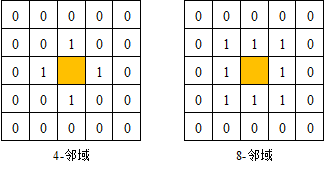常用的图像邻域分析法有两遍扫描法种子填充法。两遍扫描法会遍历两次图像，第一次遍历图像时会给每一个非0像素赋予一个数字标签，当某个像素的上方和左侧邻域内的像素已经有数字标签时，取两者中的最小值作为当前像素的标签，否则赋予当前像素一个新的数字标签。第一次遍历图像的时候同一个连通域可能会被赋予一个或者多个不同的标签。

种子填充法源于计算机图像学，常用于对某些图形进行填充。该方法首先将所有非0像素放到一个集合中，之后在集合中随机选出一个像素作为种子像素，根据邻域关系不断扩充种子像素所在的连通域，并在集合中删除掉扩充出的像素，直到种子像素所在的连通域无法扩充，之后再从集合中随机选取一个像素作为新的种子像素，重复上述过程直到集合中没有像素。

CV_EXPORTS_AS(connectedComponentsWithAlgorithm) int connectedComponents(InputArray image, OutputArray labels,
int connectivity, int ltype, int ccltype);

• image：待标记不同连通域的单通道图像，数据类型必须为CV_8U。
• labels：标记不同连通域后的输出图像，与输入图像具有相同的尺寸。
• connectivity：标记连通域时使用的邻域种类，4表示4-邻域，8表示8-邻域。
• ltype：输出图像的数据类型，目前支持CV_32S和CV_16U两种数据类型。
• ccltype：标记连通域时使用的算法类型标志，可以选择的参数及含义在表中给出该函数用于计算二值图像中连通域的个数，并在图像中将不同的连通域用不同的数字标签标记出，其中标签0表示图像中的背景区域，同时函数具有一个int类型的返回数据，用于表示图像中连通域的数目。函数的第一个参数是待标记连通域的输入图像，函数要求输入图像必须是数据类型为CV_8U的单通道灰度图像，而且最好是经过二值化的二值图像。函数第二个参数是标记连通域后的输出图像，图像尺寸与第一个参数的输入图像尺寸相同，图像的数据类型与函数的第四个参数相关。函数第三个参数是统计连通域时选择的邻域种类，函数支持两种邻域，分别用4表示4-邻域，8表示8-邻域。函数第四个参数为输出图像的数据类型，可以选择的参数为CV_32S和CV_16U两种。函数的最后一个参数是标记连通域时使用算法的标志，可以选择的参数及含义在表给出，目前只支持Grana（BBDT）和Wu（SAUF）两种算法。

OpenCV 还给出了简单的函数形式

int connectedComponents(InputArray image, OutputArray labels,
int connectivity = 8, int ltype = CV_32S);
• image：待标记不同连通域的图像单通道，数据类型必须为CV_8U。
• labels：标记不同连通域后的输出图像，与输入图像具有相同的尺寸。
• connectivity：标记连通域时使用的邻域种类，4表示4-邻域，8表示8-邻域，默认参数为8。
• ltype：输出图像的数据类型，目前支持CV_32S和CV_16U两种数据类型，默认参数为CV_32S。

该函数原型只有四个参数，前两个参数分别表示输入图像和输出图像，第三个参数表示统计连通域时选择的邻域种类，分别用4表示4-邻域，8表示8-邻域，参数的默认值为8。最后一个参数表示输出图像的数据类型，可以选择的参数为CV_32S和CV_16U两种，参数的默认值为CV_32S。该函数原型有两个参数具有默认值，在使用时最少只需要两个参数，极大的方便了函数的调用。

进一步统计每个连通域的中心位置、矩形区域大小、区域面积等信息

复杂的

CV_EXPORTS_AS(connectedComponentsWithStatsWithAlgorithm) int connectedComponentsWithStats(InputArray image, OutputArray labels,
OutputArray stats, OutputArray centroids,
int connectivity, int ltype, int ccltype);
• image：待标记不同连通域的单通道图像，数据类型必须为CV_8U。
• labels：标记不同连通域后的输出图像，与输入图像具有相同的尺寸。
• stats：含有不同连通域统计信息的矩阵，矩阵的数据类型为CV_32S。矩阵中第i行是标签为i的连通域的统计特性，存储的统计信息种类在表6-4中给出。
• centroids：每个连通域的质心坐标，数据类型为CV_64F。
• connectivity：标记连通域时使用的邻域种类，4表示4-邻域，8表示8-邻域。
• ltype：输出图像的数据类型，目前支持CV_32S和CV_16U两种数据类型。
• ccltype：标记连通域使用的算法类型标志，可以选择的参数及含义在表中给出。

该函数能够在图像中不同连通域标记标签的同时统计每个连通域的中心位置、矩形区域大小、区域面积等信息。函数的前两个参数含义与connectedComponents()函数的前两个参数含义一致，都是输入图像和输出图像。函数的第三个参数为每个连通域统计信息矩阵，如果图像中有N个连通域，那么该参数输出的矩阵尺寸为N×5，矩阵中每一行分别保存每个连通域的统计特性，详细的统计特性在表中给出，如果想读取包含第i个连通域的边界框的水平长度，可以通过stats.at(i, CC_STAT_WIDTH)或者stats.at(i, 0)进行读取。函数的第四个参数为每个连通域质心的坐标，如果图像中有N个连通域，那么该参数输出的矩阵尺寸为N×2，矩阵中每一行分别保存每个连通域质心的x坐标和y坐标，可以通过centroids.at(i, 0)和 centroids.at(i, 1) 分别读取第i个连通域质心的x坐标和y坐标。函数第五个参数是统计连通域时选择的邻域种类，函数支持两种邻域，分别用4表示4-邻域，8表示8-邻域。函数第六个参数为输出图像的数据类型，可以选择的参数为CV_32S和CV_16U两种。函数的最后一个参数是标记连通域使用的算法，可以选择的参数在上表给出，目前只支持Grana（BBDT）和Wu（SAUF）两种算法。简单的

int connectedComponentsWithStats(InputArray image, OutputArray labels,
OutputArray stats, OutputArray centroids,
int connectivity = 8, int ltype = CV_32S);
• image：待标记不同连通域的单通道图像，数据类型必须为CV_8U。
• labels：标记不同连通域后的输出图像，与输入图像具有相同的尺寸。
• stats：不同连通域的统计信息矩阵，矩阵的数据类型为CV_32S。矩阵中第i行是标签为i的连通域的统计特性，存储的统计信息种类在表6-4中给出。
• centroids：每个连通域的质心坐标，数据类型为CV_64F。
• connectivity：标记连通域时使用的邻域种类，4表示4-邻域，8表示8-邻域，默认参数值为8。
• ltype：输出图像的数据类型，目前只支持CV_32S和CV_16U这两种数据类型，默认参数值为CV_32S。

该函数原型只有六个参数，前两个参数分别表示输入图像和输出图像，第三个参数表示每个连通域的统计信息，第四个参数表示每个连通域的质心位置。后两个参数分别表示统计连通域时选择的邻域种类，分别用4表示4-邻域，8表示8-邻域，参数的默认值为8。最后一个参数表示输出图像的数据类型，可以选择的参数为CV_32S和CV_16U两种，参数的默认值为CV_32S。该函数原型有两个参数具有默认值，在使用时最少只需要四个参数，极大的方便了函数的调用。

简单示例

//
// Created by smallflyfly on 2021/6/16.
//

#include "opencv2/opencv.hpp"
#include "opencv2/highgui.hpp"

#include <iostream>

using namespace std;
using namespace cv;

int main() {

Mat gray;
cvtColor(im, gray, CV_BGR2GRAY);
//    resize(im, im, Size(0, 0), 0.5, 0.5);

imshow("im", im);

Mat im1;
threshold(gray, im1, 125, 255, THRESH_BINARY);

imshow("im1", im1);
//    waitKey(0);

RNG rng(10010);
Mat out;
int num = connectedComponents(im1, out, 8, CV_16U);
vector<Vec3b> colors;
for (int i=0; i<num; i++) {
// 使用均匀分布的随机确定颜色
Vec3b vec = Vec3b(rng.uniform(0, 256), rng.uniform(0, 256), rng.uniform(0, 256));
colors.push_back(vec);
}
Mat result = Mat::zeros(im.size(), im.type());
for (int i = 0; i < im.rows; ++i) {
for (int j = 0; j < im.cols; ++j) {
int label = out.at<uint16_t>(i, j);
if (label == 0) {
continue;
}
result.at<Vec3b>(i, j) = colors[label];
}
}

imshow("result", result);

Mat labels, stats, centroids;
int count = connectedComponentsWithStats(im1, labels, stats, centroids, 8);
cout << count << endl;

for (int i = 1; i < count; ++i) {
int x = centroids.at<double>(i, 0);
int y = centroids.at<double>(i, 1);
cout << x << " " << y << endl;
circle(im, Point(x, y), 2, Scalar(0, 0, 255), -1);
int xmin = stats.at<int>(i, CC_STAT_LEFT);
int ymin = stats.at<int>(i, CC_STAT_TOP);
int w = stats.at<int>(i, CC_STAT_WIDTH);
int h = stats.at<int>(i, CC_STAT_HEIGHT);

Rect rect(xmin, ymin, w, h);
rectangle(im, rect, Scalar(0, 255, 255), 2);
putText(im, to_string(i), Point(x+5, y), FONT_HERSHEY_SCRIPT_SIMPLEX, 1, Scalar(0, 0, 255), 2);
}

imshow("im", im);

waitKey(0);
destroyAllWindows();

return 0;

}

简单双色图效果比较明显  百度图片搜的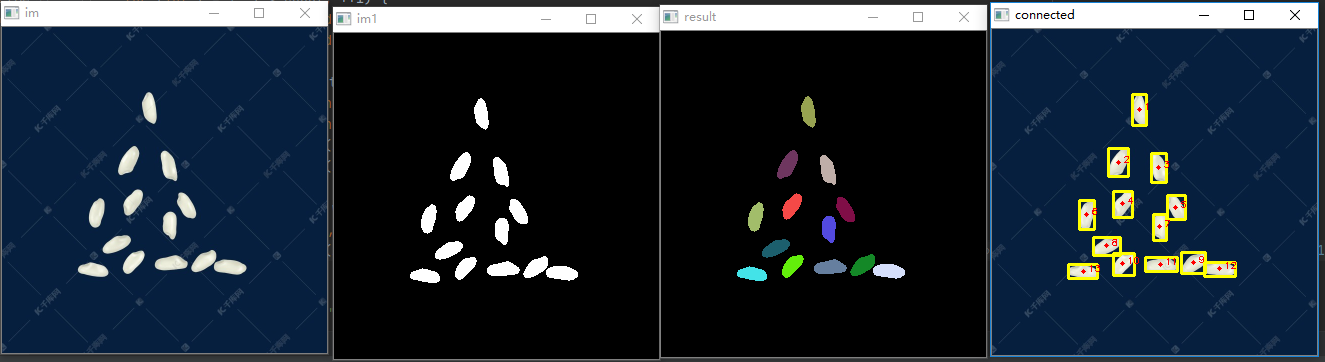展开全文opencv 图像连通域
• clear; clc; I=imread('rice.png'); s=strel('disk',2); I=imerode(I,s); I=im2bw(I); I=I*255; [m,n]=size(I); %利用队列结构 ...%指明下一个像素入队的位置 Queue=zeros(m*n,2); flag=0; for i=1:m for j=1:n
clear;
clc;
s=strel('disk',2);
I=imerode(I,s);
I=im2bw(I);
I=I*255;
[m,n]=size(I);
%利用队列结构
front=1;
rear=1;%指明下一个像素入队的位置
Queue=zeros(m*n,2);
flag=0;
for i=1:m
for j=1:n
if I(i,j)==255
Queue(rear,1)=i;
Queue(rear,2)=j;
flag=flag+1;
I(i,j)=flag;
rear=rear+1;
end
while rear~=front
temp_i=Queue(front,1);
temp_j=Queue(front,2);
front=front+1;

cond1=(temp_i-1)*(temp_j-1);
if (cond1&I(temp_i-1,temp_j-1)==255)     %1
Queue(rear,1)=temp_i-1;
Queue(rear,2)=temp_j-1;
I(temp_i-1,temp_j-1)=flag;
rear=rear+1;
end

cond2=(temp_i-1)*(temp_j);
if (cond2&I(temp_i-1,temp_j)==255)      %2
Queue(rear,1)=temp_i-1;
Queue(rear,2)=temp_j;
I(temp_i-1,temp_j)=flag;
rear=rear+1;
end

cond3=(temp_i-1)*(temp_j-n);
if (cond3&I(temp_i-1,temp_j+1)==255)     %3
Queue(rear,1)=temp_i-1;
Queue(rear,2)=temp_j+1;
I(temp_i-1,temp_j+1)=flag;
rear=rear+1;
end

cond4=(temp_i)*(temp_j-n);
if (cond4&I(temp_i,temp_j+1)==255)       %4
Queue(rear,1)=temp_i;
Queue(rear,2)=temp_j+1;
I(temp_i,temp_j+1)=flag;
rear=rear+1;
end

cond5=(temp_i-m)*(temp_j-n);
if (cond5&I(temp_i+1,temp_j+1)==255)     %5
Queue(rear,1)=temp_i+1;
Queue(rear,2)=temp_j+1;
I(temp_i+1,temp_j+1)=flag;
rear=rear+1;
end

cond6=(temp_i-m)*(temp_j);
if (cond6&I(temp_i+1,temp_j)==255)       %6
Queue(rear,1)=temp_i+1;
Queue(rear,2)=temp_j;
I(temp_i+1,temp_j)=flag;
rear=rear+1;
end

cond7=(temp_i-m)*(temp_j-1);
if (cond7&I(temp_i+1,temp_j-1)==255)     %7
Queue(rear,1)=temp_i+1;
Queue(rear,2)=temp_j-1;
I(temp_i+1,temp_j-1)=flag;
rear=rear+1;
end

cond8=(temp_i)*(temp_j-1);
if (cond8&I(temp_i,temp_j-1)==255)       %8
Queue(rear,1)=temp_i;
Queue(rear,2)=temp_j-1;
I(temp_i,temp_j-1)=flag;
rear=rear+1;
end
end
end
end

flag

初到CBIB，导师就布置了几个练习，下面是我编写的利用像素标记法实现的图像中连通域个数的统计（8邻域法）
展开全文• 连通域的原理与Python实现

千次阅读 2021-02-04 16:40:14
二值图像连通域二值图像分析最基础的也是最重要的方法之一就是连通域标记，它是所有二值图像分析的基础。它通过对二值图像中目标像素的标记，让每个单独的连通区域形成一个被标识的块，进一步的我们就可以获取这些块...

二值图像连通域

二值图像分析最基础的也是最重要的方法之一就是连通域标记，它是所有二值图像分析的基础。它通过对二值图像中目标像素的标记，让每个单独的连通区域形成一个被标识的块，进一步的我们就可以获取这些块的轮廓、外接矩形、质心、不变矩等几何参数。

连通区域的定义一般有两种，分为4邻接和8邻接。下面这幅图中，如果考虑4邻接，则有3个连通域，8邻接则是2个连通域。

从连通区域的定义可以知道，一个连通域是由具有相同像素值的相邻像素组成像素集合，因此，我们就可以通过这两个条件在图像中寻找连通区域，对于找到的每个连通域，我们赋予其一个唯一的标识( Label )，以区别其他连通域。

连通域分析的基本算法有两种： 1) Two-Pass 两遍扫描 2) Seed-Filling 种子填充法。

Two-Pass 算法

两遍扫描法( Two-Pass )，正如其名，指的就是通过扫描两遍图像，将图像中存在的所有连通域找出并标记。

(1)第一次扫描：

访问当前像素 B(x,y) ，如果 B(x,y) == 1：

a、如果 B(x,y) 的领域中标签值都为0，则赋予 B(x,y) 一个新的 label ：

label += 1， B(x,y) = label；

b、如果B(x,y)的领域中有像素值 > 1的像素Neighbors：

1)将Neighbors中的最小值赋予给 B(x,y) :

B(x,y) = min{Neighbors}

2)记录Neighbors中各个值(label)之间的相等关系，即这些值(label)同属同一个连通区域；

(2)第二次扫描：

访问当前像素 B(x,y) ，如果 B(x,y) > 1：

a、找到与 label = B(x,y) 同属相等关系的一个最小 label 值，赋予给 B(x,y) ；

完成扫描后，图像中具有相同 label 值的像素就组成了同一个连通区域。

另外，我在代码实现的过程中想到另外一种 Two-Pass 的方式(即扫描两遍图像的方式)实现，就是第二次扫描与 (1) 同样的过程,只是方向换成从右下到左上。我后面的 Two-Pass 代码是使用我自己想到的方法实现的，自己使用了几个例子测试了下，目前没出现啥问题。

Seed-Filling 算法

种子填充方法来源于计算机图形学，常用于对某个图形进行填充。它基于区域生长算法。我的理解就是递归遍历。

附上两种方法的 Python 的实现

import cv2

import numpy as np

# 4邻域的连通域和 8邻域的连通域

# [row, col]

NEIGHBOR_HOODS_4 = True

OFFSETS_4 = [[0, -1], [-1, 0], [0, 0], [1, 0], [0, 1]]

NEIGHBOR_HOODS_8 = False

OFFSETS_8 = [[-1, -1], [0, -1], [1, -1],

[-1, 0], [0, 0], [1, 0],

[-1, 1], [0, 1], [1, 1]]

def reorganize(binary_img: np.array):

index_map = []

points = []

index = -1

rows, cols = binary_img.shape

for row in range(rows):

for col in range(cols):

var = binary_img[row][col]

if var < 0.5:

continue

if var in index_map:

index = index_map.index(var)

num = index + 1

else:

index = len(index_map)

num = index + 1

index_map.append(var)

points.append([])

binary_img[row][col] = num

points[index].append([row, col])

return binary_img, points

def neighbor_value(binary_img: np.array, offsets, reverse=False):

rows, cols = binary_img.shape

label_idx = 0

rows_ = [0, rows, 1] if reverse == False else [rows-1, -1, -1]

cols_ = [0, cols, 1] if reverse == False else [cols-1, -1, -1]

for row in range(rows_, rows_, rows_):

for col in range(cols_, cols_, cols_):

label = 256

if binary_img[row][col] < 0.5:

continue

for offset in offsets:

neighbor_row = min(max(0, row+offset), rows-1)

neighbor_col = min(max(0, col+offset), cols-1)

neighbor_val = binary_img[neighbor_row, neighbor_col]

if neighbor_val < 0.5:

continue

label = neighbor_val if neighbor_val < label else label

if label == 255:

label_idx += 1

label = label_idx

binary_img[row][col] = label

return binary_img

# binary_img: bg-0, object-255; int

def Two_Pass(binary_img: np.array, neighbor_hoods):

if neighbor_hoods == NEIGHBOR_HOODS_4:

offsets = OFFSETS_4

elif neighbor_hoods == NEIGHBOR_HOODS_8:

offsets = OFFSETS_8

else:

raise ValueError

binary_img = neighbor_value(binary_img, offsets, False)

binary_img = neighbor_value(binary_img, offsets, True)

return binary_img

def recursive_seed(binary_img: np.array, seed_row, seed_col, offsets, num, max_num=100):

rows, cols = binary_img.shape

binary_img[seed_row][seed_col] = num

for offset in offsets:

neighbor_row = min(max(0, seed_row+offset), rows-1)

neighbor_col = min(max(0, seed_col+offset), cols-1)

var = binary_img[neighbor_row][neighbor_col]

if var < max_num:

continue

binary_img = recursive_seed(binary_img, neighbor_row, neighbor_col, offsets, num, max_num)

return binary_img

# max_num 表示连通域最多存在的个数

def Seed_Filling(binary_img, neighbor_hoods, max_num=100):

if neighbor_hoods == NEIGHBOR_HOODS_4:

offsets = OFFSETS_4

elif neighbor_hoods == NEIGHBOR_HOODS_8:

offsets = OFFSETS_8

else:

raise ValueError

num = 1

rows, cols = binary_img.shape

for row in range(rows):

for col in range(cols):

var = binary_img[row][col]

if var <= max_num:

continue

binary_img = recursive_seed(binary_img, row, col, offsets, num, max_num=100)

num += 1

return binary_img

if __name__ == "__main__":

binary_img = np.zeros((4, 7), dtype=np.int16)

index = [[0, 2], [0, 5],

[1, 0], [1, 1], [1, 2], [1, 4], [1, 5], [1, 6],

[2, 2], [2, 5],

[3, 1], [3, 2], [3, 4], [3, 6]]

for i in index:

binary_img[i, i] = np.int16(255)

print("原始二值图像")

print(binary_img)

print("Two_Pass")

binary_img = Two_Pass(binary_img, NEIGHBOR_HOODS_8)

binary_img, points = reorganize(binary_img)

print(binary_img, points)

print("Seed_Filling")

binary_img = Seed_Filling(binary_img, NEIGHBOR_HOODS_8)

binary_img, points = reorganize(binary_img)

print(binary_img, points)

展开全文• 在图像形态学运算中，常将不与其他区域连接的独立区域称为集合或者连通域，这个集合中的元素就是包含在连通域内的每一个像素，可以用该像素在图像中的坐标来描述，像素之间的距离可以用来表示两个连通域之间的关系....opencv
• 摘 要：针对高速图像目标实时识别和跟踪任务，需要利用系统中有限的硬件资源实现高速、准确的二值图像连通域标记，提出了一种适合FPGA实现的二值图像连通域标记快速算法。算法以快捷、有效的方式识别、并记录区域间...
• (5)根据二值图像生成的四邻域连通域 的几何形状特征...... Matlab数字图像处理-02_幼儿读物_幼儿教育_教育专区。/5、频率域图像增强 一、傅里叶变换 I=fft2(x);%快速傅里叶变换 I=fft2(x,m,n); x为输入图像;m和.......
• 做材料缺陷分析的时候，会用刀三维连通域分析这个算法，作为一个不入流的JS码农，算法我是万万不会自己去写的，而且还是用python去写。不过好在，确实有人写出了很成功的库，可以得以引用，我这里就来重点介绍一个这...
• 连通域去噪

2021-03-14 11:29:35
//计算每个标定值的像素个数 int count;for (int i = 0; i ; i++) { count[i]= 0; //初始化为0 } uchar*p =src.data;for (int i = 0; i ; i++) {for (int j = 0; j ; j++) {if (*(p + i*src.cols + j) == 0) { ...
• 4邻域：一个像素的上下左右相邻的 4个像素 8邻域：一个像素的上下左右和对角线相邻的 8个像素 4邻接：像素和它的4邻域的4个像素是4邻接的 8邻接：像素和它的8邻域的8个像素是8邻接的 满足4邻接或者8邻接的像素之间...
• matlab连通域处理函数

千次阅读 2020-12-28 21:28:17
1、 matlab函数bwareaopen──删除小面积对象 格式：BW2 = bwareaopen(BW,P,conn)...'Extent' 同时在区域和其最小边界矩形中的像素比例 'PixelIdxList' 存储区域像素的索引下标 'PixelList' 存储上述索引对应的像素坐标
• 连通域求解

千次阅读 2018-03-18 17:25:56
图像处理中连通域指由前景相同像素，并且相同像素邻接的像素组成的域。图像处理中一般都是对二值图像（1白色，0为黑色，一般前景为0黑色）做连通域分析。连通域分析指把连通域找出来并且标记出来。连通域标记方法：...图像 连通域
• 1.思路是用深度遍历，对图片进行二值化处理，先找到一个黑色像素，然后对这个像素的周围8个像素进行判断，如果没有访问过，就保存...def cfs(img):"""传入二值化后的图片进行连通域分割"""pixdata = img.load()w,h =...
• 图像的连通域是指图像中具有相同像素值并且位置相邻的像素组成的区域，连通域分析是指在图像中寻找出彼此互相独立的连通域并将其标记出来。提取图像中不同的连通域是图像处理中较为常用的方法，例如在车牌识别、文字...
• int &iConnectedAreaCount) { //拓宽1个像素的原因是：如果连通域在边缘，运行此函数会异常崩溃，所以需要在周围加一圈0值，确保连通域不在边上 //==========图像周围拓宽1个像素=================================...
• //右下 二、对某一点求连通域函数 /****************************************************************** 功能：对某个像素点，用递归函数求连通域 参数： Inimg :输入图像矩阵 Flag :像素点有没有遍历过标志 0 ...
• 连通域分析其实是一个路径搜索问题，搜索方式就看...对他进行连通域搜寻，将搜寻到的整个连通域内的像素点标为2(为了避免与本来的颜色1冲突)继续搜索像素值为1的点(之前联通域分析过的已经将像素值改为大于1的值，...
• OpenCV—python 连通域标记

千次阅读 2020-12-18 15:01:34
图像的连通域是指图像中具有相同像素值并且位置相邻的像素组成的区域，连通域分析是指在图像中寻找出彼此互相独立的连通域并将其标记出来。提取图像中不同的连通域是图像处理中较为常用的方法，在目标检测等领域对感...opencv
• //=======调用函数===================================================================== 以上这篇使用OpenCV去除面积较小的连通域就是小编分享给大家的全部内容了，希望能给大家一个参考，也希望大家多多支持...
• 通过以上函数，确定出二值图片中的一些连通域，接下来通过一些筛选条件，即连通域宽高比的无用连通域过滤掉，想把无用的连通域设置为背景色（黑色）。请问如何将这些区域设置为黑色？？？？？？ 已有思路：得到的...人工智能 自然语言处理
• 基于opencv的cv::connectedComponentsWithStats()的连通域分析标记算法： 一、函数介绍： 在OpenCV3中有了新的专门的函数 cv::connectedComponents() 和函数 cv::connectedComponentsWithStats(); 来做连通域分析，...c++ 算法 opencv
• 二值图像在图像分析与识别中有着举足轻重的地位，因为其模式简单，对像素在空间上的关系有着极强的表现力。在实际应用中，很多图像的分析最终都转换为二值图像的分析，比如：医学图像分析、前景检测、字符识别，形状...
• 连通域分析

千次阅读 2019-05-23 21:48:11
连通域分析是指找出图中图像中的各个连通区域并标记。 连通域分析存在两种基本算法，Two-Pass算法，Seed-Filling算法。 Two-Pass算法 目标图像为二值化图像，前景像素为255，背景像素为0 第一遍扫描 访问当前...
• 上一篇我们讲到了MATLAB中的bwlabel连通域标记...简单点说就是每次以一个需要标记的像素点为种子，然后不断向其周围扩散，找出其他的与其相连通的可标记的像素点，这样就能标记出一个连通域，然后再以另一个连通域...连通域 C opencv 图像处理 深度优先搜索
• 分水岭分割算法是一个经典的分割算法，其基本原理把图像看作是测地学上的拓扑地貌，图像中每一点像素的灰度值表示该点的海拔高度，每一个局部极小值及其影响区域称为集水盆，而集水盆的边界则形成分水岭。...分水岭分割...